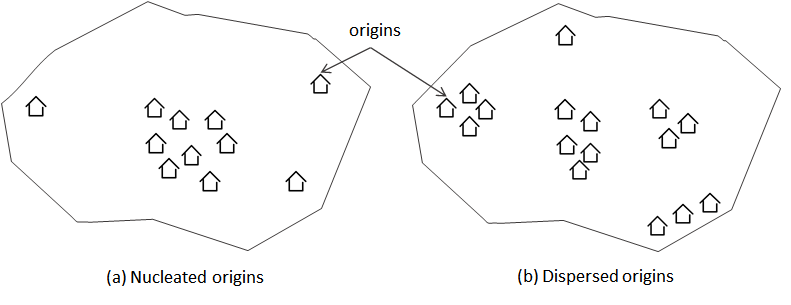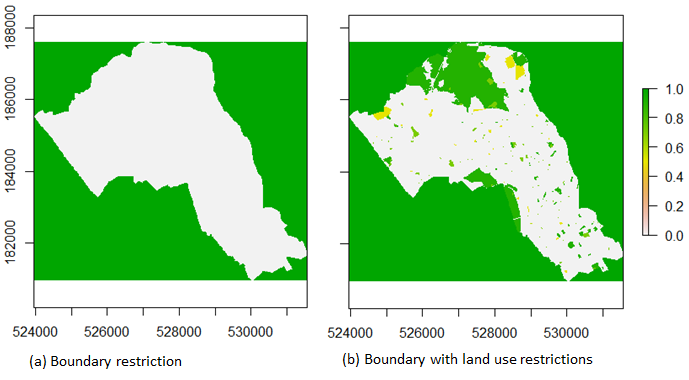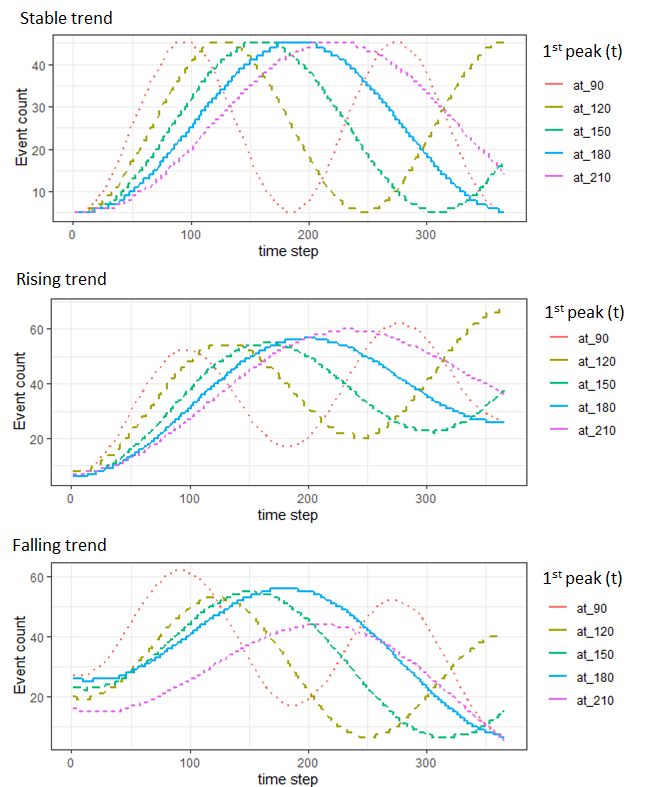## Introduction

In many research contexts, access to fine-grained spatiotemporal (ST) point data have been severely restricted due to privacy concerns. The R-stppSim package has been designed to address this challenge by presenting a framework that is capable of mimicking a real-life data, through the integration of microsimulation and agent-based techniques, in order to generate new point events in space and time. The framework comprises a set of ‘walkers’ (agents, objects, persons, etc.) with modifiable movement characteristics, the landscape (spatial components), and the temporal configurations. It is The interaction between these three elements that give rise to new point events, which can be processed, measured, and manipulated for further applications.

The package contains two key functions for synthesizing new datasets; (i) psim_artif and (ii) psim_real. The psim_artif synthesizes point patterns purely from users specifications (simulation from scratch). This implies that the simulation does not depend on any existing point data. On the other hand, psim_real synthesizes point patterns based on a sample real data set. The latter is particularly applicable to situations where only sparse version of a data set is available for an area. The function learns (or extracts) certain spatial and temporal characteristics of the sample data, and then extrapolates to generate full data set. The potential applications of stppSim include generation of offending data sets (crime), disease infection data sets, and foraging point patterns of wild animals.

## Simulation Parameters

The simulation parameters are in relation to three elements, namely; the ‘walkers (agents)’, the landscape (spatial), and the temporal dimension. The parameters are described as follow:

### Walkers (agents)

The walkers are defined primarily by the following characteristics:

• Movement - Walkers can move in any direction and have the ability to detect obstructions (restrictions) on their path. The movements are controlled primarily by an in-built transition matrix (TM) which defines two operational states, namely; an exploratory state (in which a walker is merely exploring the environment) and a performative state (in which a walker is doing an action). The stochastic properties of the TM ensure variations in the behavioral patterns amongst walkers. In order to switch from one state to another, a categorical distribution is assigned to the latent state variable $$z_{it}$$. So, every time step may be assigned to either operational state, independent of the previous state: $z_t \sim Categorical(\Psi{_{1t}}, \Psi{_{2t}})$ Such that $$\Psi{_{i}}$$ = Pr$$(z_t = i)$$, where $$\Psi{_{i}}$$ is the fixed probability of being in state $$i$$ at time $$t$$, and $$\sum_{i=1}^{z}\Psi{_{i}}=1$$

• Spatial perception [s_threshold] - Perception range of a walker at a given location. The parameter s_threshold is generally updated as a walker moves to a new location. A natural method for choosing this parameter is to plot out the data, choose the estimate that is most in accordance with one’s prior ideas about the s_threshold value. For many applications this approach will be perfectly satisfactory. For psim_artif, a user will be expected to define a value, but for psim_real, the optimal s_threshold value may be estimated based on the sample data set.

• Steps [step_length] - The maximum step taken by a walker from one point location to the next. This defines the speed of a walker across the space. The step_length should be carefully defined, particularly, when movements are restricted along narrow paths, such as a route network. In this case, the value must be smaller than the width of the paths.

• Proportional ratios [p_ratio] - Defines the spatial concentration of events being generated by the walkers. Defined as the percentage of total events emanating from a small number of the most active origins. For example, a 20:80 proportional ratios implies that 20% of origins (walkers) would generate 80% of the total point events. In other words, origins have strength values which can be utilized to model the expected (final) spatial distribution of events (points), named the spatial model.

### Landscape (spatial)

The followings are the key properties of a landscape:

• Origins [coords] - Walkers emanate from origins. Origins may be distributed randomly across the landscape or exhibit specific spatial concentration. Origins are defined in terms of xy coordinates. In criminological application, a human offender can be modelled as a walker emanating from his residence (origin). Origins can exhibit two types of concentration: nucleated and dispersed (Hornby and Jones, 1991). A nucleated concentration is one in which all origins concentrate around one focal location, while a dispersed concentration has multiple focal locations and the origins can be completely random across the space (see fig. 1).Figure 1: Type of origin concentration

• Boundary [poly] - A landscape is bounded - defined by a polygon shapefile (poly) or by the spatial extent of the sample point data.

• Restrictions [restriction_feat] - Features constituting obstructions. Comprising two parts: (i) Areas outside the boundary (poly) with maximum restriction value of 1. That is, walkers can not step outside the boundary. (ii) Features within the boundary serving as obstructions to movement, e.g., certain land use type or land features, such as a fenced place and hills. Typically, to generate a restriction map, two steps are involved. Using the example of boundary shapefile of Camden area of London (UK), a restriction map can be generated as follows:

Step 1: Generate boundary restriction

#load shapefile data
#extract boundary shapefile
boundary = camden$boundary # get boundary #compute the restriction map restrct_map <- space_restriction(shp = boundary,res = 20, binary = TRUE) #plot the restriction map plot(restrct_map) Step 2: Setting the restrct_map above as the basemap, and then stack the land use features to define the restrictions within the area, # get landuse data landuse = camden$landuse

#compute the restriction map
full_restrct_map <- space_restriction(shp = landuse,
baseMap = restrct_map, res = 20, field = "restrVal", background = 1)

#plot the restriction map
plot(full_restrct_map)Figure 2: Restriction map

From figure 2, the land use feature has three classes, with each class having it’s own restriction value {e.g., Leisure (0.5); Sports (0.7); and Green (0.9)},

• Focal points [n_foci] - Locations (origins) of relatively higher importance. Usually present more opportunities (to event occurrences). This is only specified when using psim_artif. A user will typically specify the number of focal points to simulate. In the context of urban landscape configuration, a focal point is synonymous to a city/town centre. The major focal point (location) within a city (if exist) can also be specified, using an additional parameter mfocal. The default value of mfocal is NULL. Also, a parameter foci separation allows a user to specify the proximity of the focal points to one another (values range from 1 to 100), with 1 and 100 being highest and lowest proximities, respectively.

### Temporal dimension

The following parameters define the temporal dimension:

• Long-term trend [trend] - Defines the long-term direction of the time series to be simulated. The can be stable, rising or falling. When rising or falling, an additional slope argument can be used to specify whether the trend slope is gentle or steep. Also, only applies to simulation from scratch.

• Seasonal patterns [first_pDate] - Defines the medium-term fluctuations of the total events over time. This is controlled by specifying the first seasonal peak point of the time series. A 90 day first peak implies a seasonal cycle of 180 days.Figure 3: Global trends and patterns

Figure 3 shows expected seasonal patterns based on different values of first_pDate, starting with 90 days, and then increasing the value successively by one month. The number of seasonal cycles decreases with later first_pDate values. Both the long-term trend and the seasonal patterns are learned when simulating using psim_real.

The combination of the long-term trend and the seasonal patterns represents the temporal model of a simulation. This should be previewed/review before starting the simulation.

• time bin - Time to reset all walkers. Typically 1 day.

## Installation of stppSim

From R console, type:

#To install from  CRAN
install.packages("stppSim")

#To install the developmental version, type:
remotes::install_github("MAnalytics/stppSim")
#Note: remotes is an extra package that needed to be installed prior to the running of this code.

library(stppSim)

## Important Information:

### Using interactive argument

Both psim_artif and psim_real functions have the argument interactive which is set as FALSE (by default). If the interactive argument is set as TRUE, then queries are printed in the console (when running the functions) asking the user whether to show the spatial and temporal models of the simulation. The spatial model shows the location of the origins as well as their strength distribution across the space. The strength distribution represents the likeness of the point (event) distribution to be simulated. Similarly, the temporal model shows the trend and seasonal pattern (smoothened) to be expected from the simulation. Therefore, a user has the opportunity to review both the spatial and the temporal patterns before proceeding with the simulation.

## Simulating stpp from scratch

Three key arguments required are: n_events - the number of points to simulate, start_date - the start date of the time series, and poly - the polygon shapefile representing the boundary of the study area within which point patterns are to be simulated. For the former, it is recommended that a vector of values is provided, rather than a single value. For example, n_events = c(200, 500, 1000, 2000). The output is generated as a list comprising the separate data frame for each value. Besides, the length of n_events has little of no effects on the processing time.

### Example

Given the boundary shapefile of Camden Borough of London (embedded in the package), the stpp can be generated as follows:


boundary <- camden$boundary # get boundary data #specifying data sizes pt_sizes = c(200, 1000, 2000) #simulate data artif_stpp <- psim_artif(n_events=pt_sizes, start_date = "2021-01-01", poly=boundary, n_origin=50, resistance_feat = NULL, field = NA, n_foci=5, foci_separation = 10, mfocal = NULL, conc_type = "dispersed", p_ratio = 20, s_threshold = 50, step_length = 20, trend = "stable", first_pDate=NULL, slope = NULL,show.plot=FALSE, show.data=FALSE)  The processing time on an Intel Core i7-7500CPU @ 2.70GHz, 16.0GB RAM PC is 3.5 minutes. The processing time is increases to 30.2 minutes if landscape restriction is added. That is, if the argument resistance_feat = camden$landuse (with field = "restrVal").
To retrieve the result of any n_events, simply type the object name with the value index. For example to retrieve the result based on n_events = 1000, type:
stpp_1000 <- artif_stpp[]
The spatial patterns and clustering of events can be controlled by manipulating the arguments that control the properties of the spatial components (e.g., resistance_feat, n_origin, mfocal, foci_separation, n_foci, etc.) and the ones that control the properties of the walkers (e.g. step_length, s_threshold, p_ratio). Specifically, in order to add a focal location to the simulation (i.e. mfocal - see explanation above), use the make_grids function to generate an interactive map from which the xy coordinates of every location in the map can be displayed/extracted. The interactive map includes an OpenStreetMap that allows a user to identify places more easily.
Figure 4 is the spatial point patterns (spp) of n_events = 1000 at varying parameter settings. Note: the spatial pattern is likely to change each time the code is re-run, owing to the random elements in the function. Figure 4a is the result when all default arguments are used (as in the code above). Figure 4b is the result when the following extra arguments are applied, namely; resistance_feat = camden\$landuse and mfocal = c(530000, 182250) - the first argument limits the amount of events simulated within the land use (restriction) features, and the second argument ensures that the spatial concentration of origins are around a focal point (point indicated as red dot on the map). Figure 4c is the result when, in addition to applying the resistance_feat and mfocal (as above), the foci_separation = 50 - this additional argument ensures that the origins are moderately far from each another. Lastly, Figure 4d is the result when, in addition to setting the mfocal (as above), the s_threshold and step_length are set as equal to 250 and 50, respectively - these additional settings is to ensure that points are well-spread out from their respective origins.